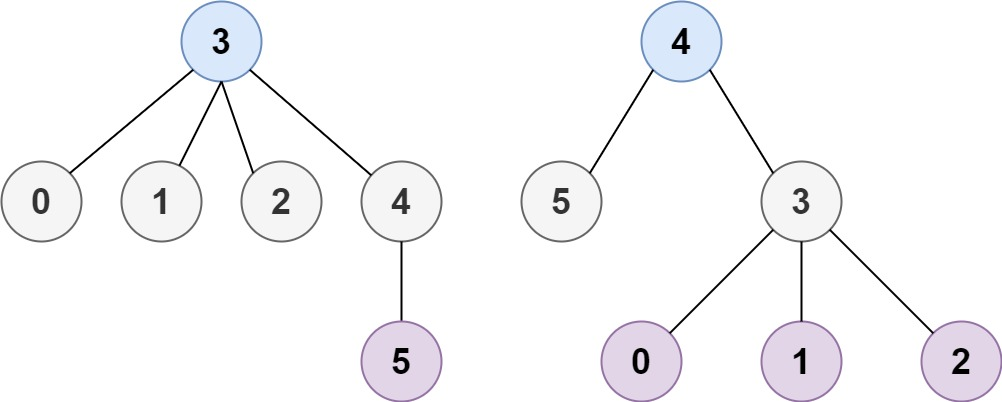# Minimum Height Trees LeetCode Solution

Difficulty Level Medium

## Problem Statement

Minimum Height Trees LeetCode Solution – We are given a tree of n nodes labelled from 0 to n-1 as a 2D array “edges” where edge[i] = [a_i, b_i] indicates that there is an undirected edge between the two nodes a_i and b_i in the tree. We have to select a node x as the root, the result tree has height h. Among all possible rooted trees, those with minimum height are called minium height trees (MHTs). We are asked to return a list of all MHTs’ root labels.

The height of a rooted tree is the number of edges on the longest downward path between the root and a leaf.

## Examples & Explanation

Example 1:```Input: n = 4, edges = [[1,0],[1,2],[1,3]]
Output: 
Explanation: As shown, the height of the tree is 1 when the root is the node with label 1 which is the only MHT.```

Example 2:```Input: n = 6, edges = [[3,0],[3,1],[3,2],[3,4],[5,4]]
Output: [3,4]
Explanation: we get the minimum height as 2 when node 3 and 4 are selected as roots & hence the answer is [3,4]```

## Approach

The most obvious intuition that comes to mind is to apply the shortest path algorithm to find the distance between the root node to all other nodes. This approach requires applying Dijkstra from all nodes. We can keep a track of which length was the shortest and what root nodes lead to the shortest paths. This algorithm takes a considerable amount of time. Can we do better?

If we observe closely, the roots of MHT must be the midpoints of the longest leaf to leaf path in the tree. To obtain middle nodes, we can slice out all the outermost nodes till we are left with one or two nodes. As the slicing is done layer-wise, from outermost to innermost (till one or 2 is(are) left) it is also called the ripple effect. The diagram might give you a better idea about this algorithm.We can clearly see how layers begin sliced out one by one till the middle nodes are left.

Let’s use Kahn’s algorithm for topo sort and BFS ripple effect to make the algorithm efficient.

To apply these algorithms, create an undirected graph and maintain an array “degree” to store the number of edges linked to a node. Create a queue and push all the nodes that are present in the outermost layer i.e. degree having 1.

Run BFS ripple effect: run a while loop “sz” times, where sz = size of the queue. Delete the front elements of the queue and store them in a separate array or vector and push all its neighbors into the queue if they become the part of the next outermost layer, i.e have a degree of 1.

When the queue becomes empty, the array used for storing sliced-out elements will contain middle nodes and hence the answer.

## Code

### C++ code for Minimum Height Trees LeetCode Solution

```class Solution {
public:
vector<int> findMinHeightTrees(int n, vector<vector<int>>& edges) {
if(n==0) return {};
if(n==1) return {0};
if(n==2) return {0,1};
vector<int> degree(n,0);
vector<int> res;
for(auto &edge: edges) {
degree[edge]++;
degree[edge]++;
}
queue<int> q;
for(int i=0; i<n; i++) {
if(degree[i] == 1) {
q.push(i);
}
}

while(!q.empty()) {
vector<int> temp;
int sz = q.size();

while(sz--) {
int u = q.front();
q.pop();
temp.push_back(u);

if(--degree[v] == 1) {
q.push(v);
}
}
}
res=temp;
}
return res;
}
};```

### Java code for Minimum Height Trees LeetCode Solution

```class Solution {
public List<Integer> findMinHeightTrees(int n, int[][] edges) {
if (n < 2) {
ArrayList<Integer> res = new ArrayList<>();
for (int i = 0; i < n; i++)
return res;
}

Map<Integer, List<Integer>> adj = new HashMap();
int[] deg = new int[n];
List<Integer> res = new ArrayList();

for(int[] edge: edges){
}

}

deg[edge]++;
deg[edge]++;
}

for(int i=0; i<n ; i++){
}

while(!q.isEmpty()){
List<Integer> temp = new ArrayList();
int sz = q.size();

while(sz-- > 0){
int u = q.remove();

}
}

res = temp;
}

return res;
}
}```

## Complexity Analysis for Minimum Height Trees LeetCode Solution

Let |V| = the number of nodes in the graph, then the number of edges would be |E| = |V| – 1

• Time Complexity: O(|V|)
• Time to construct graph = |E| iterations
• BFS to slice out layers will take |E|+|V| = |V|+|V|-1
• Overall time complexity is O(|V|)
• Space Complexity: O(|V|)
• Adj map takes V+E space i.e. O(|V|)
• Defined queues, arrays, or vectors can have at max V numbers
• Overall space complexity is O(|V|)
Translate »